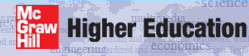# Institut für Thermodynamik der Luft- und Raumfahrt - Universität StuttgartMechanical Engineering - The University of Texas at Austin# units

TEXSTAN works in dimensional variables that must adhere to either the U.S. Customary Units convention (often called the British or English unit system) or the SI units convention (often called the metric unit system).

kunits units for all dimensional input variables to TEXSTAN
=0 U.S. Customary (British or English) units system
=1 SI (metric) units system

To permit this flexibility of units, there are two conversion factors built into all equations within TEXSTAN (where needed). The first is the force-mass conversion factor from Newton's Second Law, gc , and the second is the work energy-heat energy conversion factor from the First Law of Thermodynamics, J. These factors are programmed into the equations as the FORTRAN variables gc and cj.

• U.S. Customary Units conversion factors (kunits=0)
• gc = 32.1740 (lbm-ft/s2)/lbf
• cj = 778.16 (lbf-ft)/Btu
• SI Units conversion factors (kunits=1)
• gc = 1.0 (kg-m/s2)/N
• cj = 1.0 (N-m)/J

Note that students in the SI world rarely need to explicitly consider these issues because the numerical calculations rarely depend on these conversion factors, partly because force and mass are not independently defined like they are in U.S. Customary (British-English) units, and partly because work and heat often share similar units, whereas they have totally different units in the U.S. Customary (British-English) system.

When U.S. Customary (British-English) units are being considered for the input variables, then all input variables must adhere to the following convention:

• force: lbf (pounds-force)
• mass: lbm (pounds-mass)
• length: ft (foot)
• time: s (second)
• energy: Btu (British thermal unit)

For temperature either °F or °R must be used. Note that it is tempting to adopt other "convenient units" such as inches rather than feet (ft), or minutes or hours rather than seconds (s), but this is not permitted in TEXSTAN. Only the units that appear in the conversion factors can be used in forming the input variables.

When SI (metric) units are being considered for the input variables, then all input variables must adhere to the following convention:

• force: N (Newton)
• mass: kg (kilogram)
• length: m (meter)
• time: s( second)
• energy: J (Joule)

For temperature either °C or K must be used. Note that it is tempting to adopt other "convenient units" such as centimeters or millimeters rather than meters (m), or grams rather than kilograms (kg), but this is not permitted in TEXSTAN. Only the units that appear in the conversion factors can be used in forming the input variables.

Another very important consideration when SI (metric) is chosen as the units base: the energy unit is Joules and not kilojoules. This is especially important for the specific heat specification when constant properties are being considered.

The following is a summary of the U.S. Customary units and SI units for variables used in TEXSTAN

unit U.S. Customary SI
force lbf N
length ft m
temperature °F or °R °C or K
time s s
density lbm/ft3 kg/m3
energy Btu J (not kJ)
specific heat Btu/(lbm-°R) J/(kg-K)
enthalpy Btu/lbm J/kg (not kJ/kg)
gravity constant ft/s2 m/s2
heat flux Btu/(s-ft2) J/(s-m2) (or watts/m2)
power Btu/s J/s (or watts)
mass flux lbm/(s-ft2) kg/(s-m2)
pressure lbf/ft2 N/(m2) (or Pa)
velocity ft/s m/s
viscosity (dynamic) lbm/(s-ft) (N-s)/m2 (or Pa-s)

website updated Sept 2010   © 1996-2010 Michael E. Crawford - all rights reserved - website validated for CSS 2.1 and XHTML 1.0 strict at www.w3.org